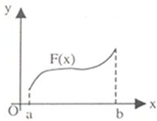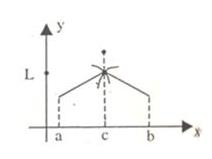Courses

Revision Notes - Continuity Notes | Study Mock Test Series for JEE Main & Advanced 2022 - JEE

JEE: Revision Notes - Continuity Notes | Study Mock Test Series for JEE Main & Advanced 2022 - JEE

The document Revision Notes - Continuity Notes | Study Mock Test Series for JEE Main & Advanced 2022 - JEE is a part of the JEE Course Mock Test Series for JEE Main & Advanced 2022.
All you need of JEE at this link: JEE
• A function f(x) is said to be continuous at x= a if
limx→a- f(x)= limx→a+ f(x)=f(a)
Thus, unlike limits, for continuity it is essential for the function to be defined at that particular point and the limiting value of the function should be equal to f(a).
• The function f(x) will be discontinuous at x =a in either of the following situations:
1. limx→a- f(x) and limx→a+ f(x) exist but are not equal.
2. limx→a- f(x) and limx→a+ f(x) exist and are equal but not equal to f(a).
3. f(a) is not defined.
4. At least one of the limits does not exist.
• If you are required to comment on the continuity of a function, then you may just look for the points on the domain where the function is not defined.

Some important properties of continuous functions:
If the functions f(x) and g(x) are both continuous at x =a then the following results hold true:
1. cf (x) is continuous at x =a where c is any constant.
2. f(x) + g(x) is continuous at x = a.
3. f(x).g(x) is continuous at x= a.
4. f(x)/g(x) is continuous at x= a, provided g(a) ≠ 0.

• If a function f is continuous in (a, b), it means it is continuous at every point of (a, b).
• If f is continuous in [a, b] then in addition to being continuous ay every point of domain, f should also be continuous at the end points i.e. f(x) is said to be continuous in the closed interval [a, b] if
1. f(x) is continuous in (a, b)
2. limx→a+ f(x)=f(a)
limx→a- f(x)=f(a)
• While solving problems on continuity, one need not calculate continuity at every point, in fact the elementary knowledge of the function should be used to search the points of discontinuity.
• In questions like this where a function h is defined ash(x) = f(x) for a < x < b
g(x) for b < x < c
The functions f and g are continuous in their respective intervals, then the continuity of function h should be checked only at the point x = b as this is the only possible point of discontinuity.
• If the point ‘a’ is finite, then the necessary and sufficient condition for the function f to be continuous at a is that limx→a- f(x) and limn→a+ f(x) should exist and be equal to f(a).
• A function continuous on a closed interval [a, b] is necessarily bounded if both a and b are finite. This is not true in case of open interval.
• If the function u = f(x) is continuous at the point x=a, and the function y=g(u) is continuous at the point u = f(a), then the composite function y=(gof)(x)=g(f(x)) is continuous at the point x=a.
• Given below is the table of some common functions along with the intervals in which they are continuous: Functions f(x) Interval in which f(x) is continuous Constant C (-∞,∞) bn, n is an integer > 0 (-∞,∞) |x-a| (-∞,∞) x-n, n is a positive integer. (-∞,∞)-{0} a0xn + a1xn-1 +........ + an-1x + an (-∞,∞) p(x)/q(x), p(x) and q(x) are polynomials in x R - {x:q(x)=0} sin x R cos x R tan x R-{nπ:n=0,±1,........} cot x R-{(2n-1)π/2:n=0,±1,± 2,........} sec x R-{(2n-1)π/2:n=0,±1,± 2,........} ex R ln x (0, ∞)

If you know the graph of a function, it can be easily judged without even solving whether a function is continuous or not. The graph below clearly shows that the function is discontinuous.

• If limx→af(x) = L1 and limx→a+ f(x) = L2, where L1 and Lare both finite numbers then it is called discontinuity of first kind or ordinary discontinuity.• A function is said to have discontinuity of second kindif neither limx→af(x) nor limx→a- f(x) exist.
• If any one of limx→a+ f(x) or limx→a- f(x) exists and the other does not then the function f is said to have mixed discontinuity.
• If limx→a f(x) exists but is not equal to f(a), then f(x) has removable discontinuity at x = a and it can be removed by redefining f(x) at x=a.
• If limx→a f(x) does not exist, then we can remove this discontinuity so that it becomes a non-removable or essential discontinuity.
• A function f(x) is said to have a jump discontinuity at a point x=a if, limx→a-f(x) ≠ limx→a+ f(x) and f(x) and may be equal to either of previous limits.
The concepts of limit and continuity are closely related. Whether a function is continuous or not can be determined by the limit of the function.
The document Revision Notes - Continuity Notes | Study Mock Test Series for JEE Main & Advanced 2022 - JEE is a part of the JEE Course Mock Test Series for JEE Main & Advanced 2022.
All you need of JEE at this link: JEEUse Code STAYHOME200 and get INR 200 additional OFF Use Coupon Code

Top Courses for JEEMock Test Series for JEE Main & Advanced 2022

2 videos|258 docs|160 tests

Top Courses for JEETrack your progress, build streaks, highlight & save important lessons and more!

,

,

,

,

,

,

,

,

,

,

,

,

,

,

,

,

,

,

,

,

,

;